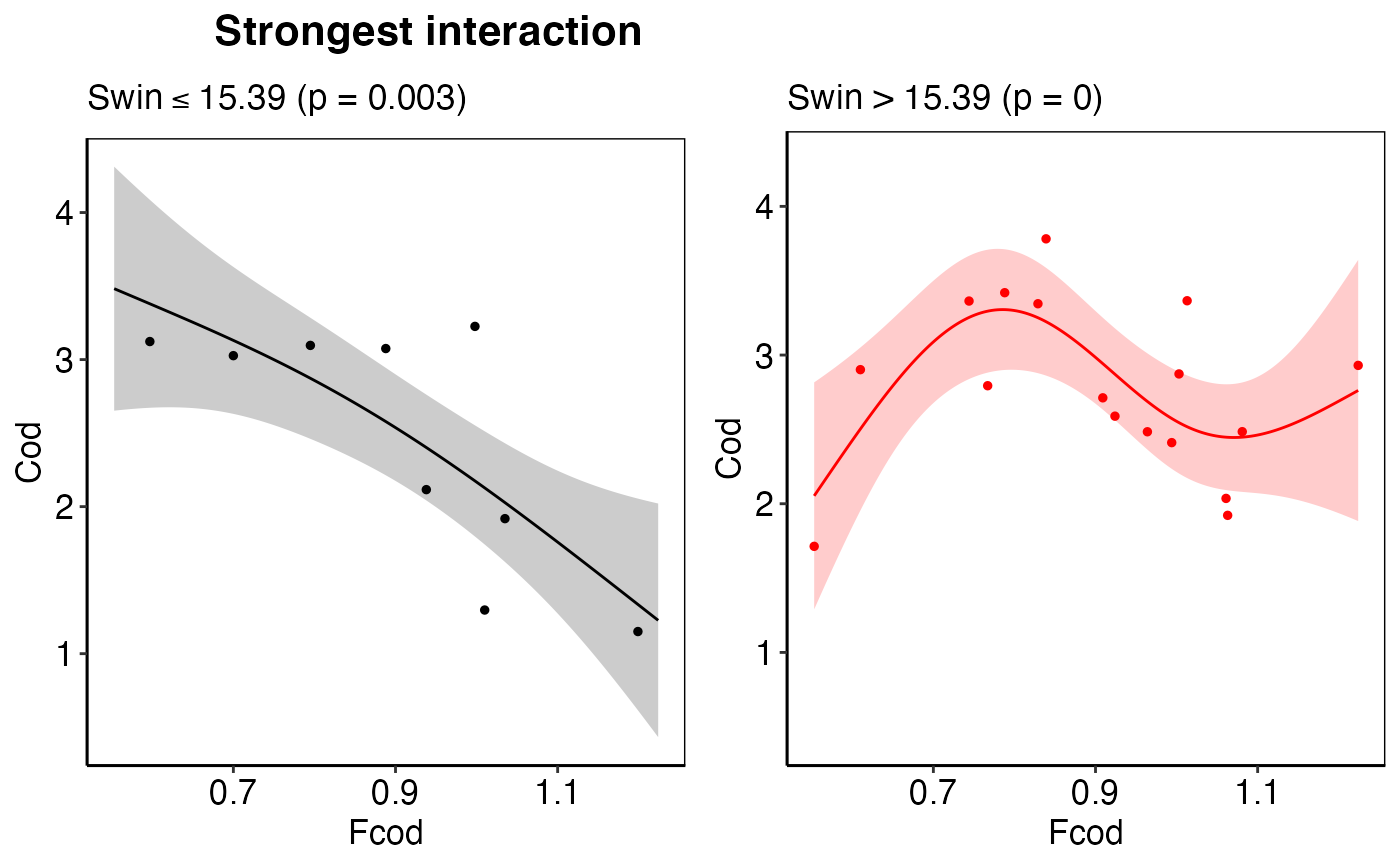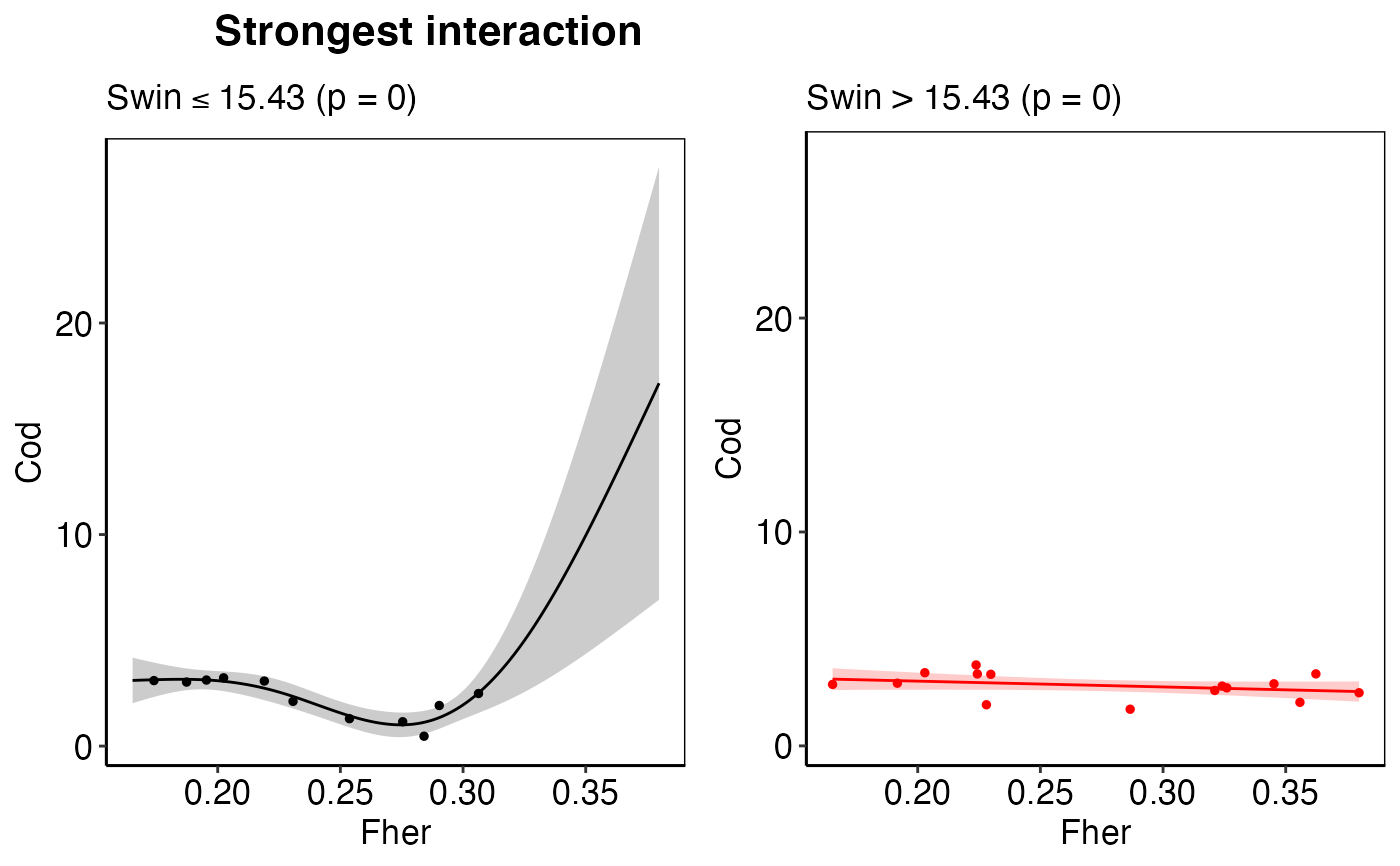These are the actual plotting functions for each of the 4 plots shown in the wrapper function plot_model. plot_thresh works currently only for threshold-GAMs but not for threshold_GAMMs.

plot_thresh(thresh_sublist, choose_thresh_gam)

plot_response(
x,
y,
x_seq,
pred,
ci_up,
ci_low,
xlab,
ylab,
pos_text,
label,
title = "Response curve S"
)

plot_predict(
x,
y_obs,
y_pred,
ci_up,
ci_low,
x_train,
x_test,
zoom,
x_range,
y_range,
xlab,
ylab,
pos_text,
label,
title = "Predictive performance"
)

plot_deriv(
press_seq,
deriv1,
deriv1_ci_low,
deriv1_ci_up,
zic_start_end,
zero_in_conf,
xlab,
ylab,
pos_text,
label
)

plot_all_mod(p1, p2, p3, p4, title)

plot_model

## Examples

# Example for dealing with nested list-columns using the
# Baltic Sea demo data
thresh_sublist1 <- all_results_ex$thresh_models[] thresh_sublist2 <- all_results_ex$thresh_models[]
thresh_sublist <- list(thresh_sublist1, thresh_sublist2) %>%
purrr::flatten(.)
plot_thresh(thresh_sublist, choose_thresh_gam = NULL)plot_thresh(thresh_sublist, choose_thresh_gam = 1)plot_thresh(thresh_sublist, choose_thresh_gam = 2)